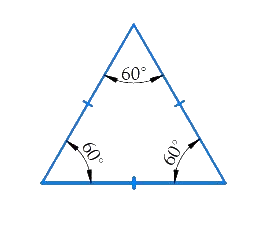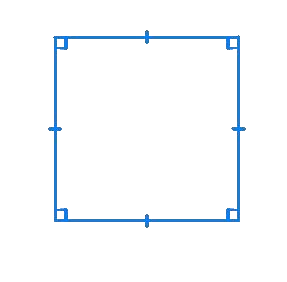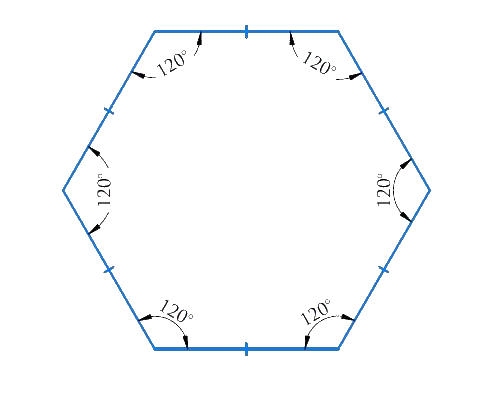# Ex.3.1 Q5 Understanding Quadrilaterals Solution - NCERT Maths Class 8

Go back to  'Ex.3.1'

## Question

What is a regular polygon? State the name of a regular polygon of

(i) $$3$$ sides

(ii) $$4$$ sides

(iii) $$6$$ sides

Video Solution
Ex 3.1 | Question 5

## Text Solution

What is known?

Number of sides of polygon

What is unknown?

Name of a regular polygon of

(i) $$3$$ sides

(ii) $$4$$ sides

(iii) $$6$$ sides

Steps:

Regular polygon - A polygon having all sides of equal length and the interior angles of equal measure is known as regular polygon i.e. a regular polygon is both ‘equiangular’ and ‘equilateral

(i) $$3$$ sides $$=$$ polygons having three sides is called a Triangle.(ii) $$4$$ sides $$=$$ polygons having four sides is called square/quadrilateral.(iii) $$6$$ sides $$=$$ polygons having six sides is called a HexagonLearn from the best math teachers and top your exams

• Live one on one classroom and doubt clearing
• Practice worksheets in and after class for conceptual clarity
• Personalized curriculum to keep up with school